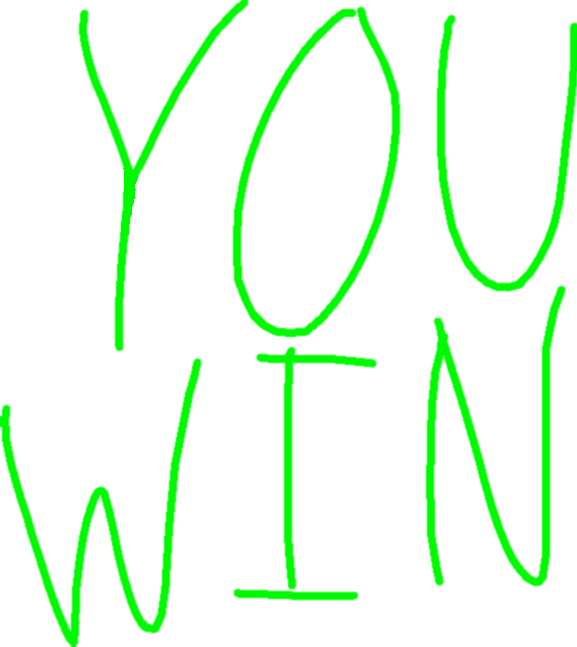# Quiz Guy

142
10146
Share
Report

### Description

Quiz Guy, a project made by Irritating Camp using Tynker. Learn to code and make your own app or game in minutes.

### Tags

Educational, Quiz, History, Art, Stick Figure, Game, Trivia, Storytelling, Virtual Friend, Math

### Concepts

basic math, miscellaneous, simple conditionals, simple variables, variables, delays, simple sound playing

• #Lines:453
• #Actors:4
• #Costumes:6
• #Scripts:9

### Text Snippets

• would you like a quiz?
• what is your name, player?
• which subject would you like?
• american history
• amercian history
• when was the decloration of indepenance signed?
• i'm sorry, but the answer is 1776.
• who was the 16th president?
• theodore roosevelt
• george washington
• i'm sorry, but the answer os abraham lincoln
• when did the u.s. enter world war ii?
• i'm sorry, but the answer is 1941.
• when did the revolutionary war end?
• i'm sorry, but the answer is 1783.
• who made the louisana purchase?
• thomas jefferson
• i'm sorry, but the answer is thomas jefferson.
• who was the soviet union fighting against in the cold war?
• the united states of america
• i'm sorry, but the answer the united states of america.
• why did richard nixon resign?
• cuban missile stave
• watergate scandal
• i'm sorry, but the answer is the watergate scandal.
• who assassinated john f. kennedy?
• lee harvey oswald
• dwight david eisenhower
• john wilkes booth
• i'm sorry, but the answer is lee harvey oswald.
• which apollo mission got man on the moon?
• i'm sorry, but the answer is apollo 11.
• i have no more questions for you.
• what does baking soda and vinegar make when you mix them?
• i'm sorry, but the answer is an eruption.
• what is the lightest atom?
• i'm sorry, but the answer is hydrogen.
• what is sand made of?
• i'm sorry, but the answer is so2.
• why are plants green?
• i'm sorry, but the answer is chlorophyll.
• who made the periodic table?
• dmitri mendeleev
• i'm sorry, but the answer is dmitri mendeleev.
• what is the densest thing in the universe?
• i'm sorry, but the answer is neutron star.
• which is the brightest: a blazar, a quazar or a kilonova?
• i'm sorry, but the answer is quazar.
• how much of the earth's surface is water?
• i'm sorry, but the answer is 71%.
• if hydrogen is the fuel for stars, what is its ash?
• i'm sorry, but the answer is iron.
• what is (3x4)+(8-4)?
• i'm sorry, but the answer is 16.
• what is 7x8+(9-3)
• i'm sorry, but the answer is 62.
• i'm sorry, but the answer is 48.
• if the raduis of a circle is 27, what is its circumfrance?
• i'm sorry, but the answer is 169.56.
• which list has like terms? (note that a digit at the end of a variable means 'power to said digit').
• i'm sorry, but the answer is 4y6 9y6.
• solve (3a+5b)-c for a=8 b=2 & c=9
• i'm sorry, but the answer is 25.
• if two sides of a right triangle that aren't the hypotenuse are 16 and 12, what is the area?
• i'm sorry, but the answer is 96.
• which comes next after the following: 1 1 2 3 5 8 13 21 34
• i'm sorry, but the answer is
• which is irrational?
• square root of 2
• i'm sorry, but the answer is square root of 2
• (if) two plus two is four, (what's) minus one?
• everyday's man of the block
• although black holes can be considered the densest thing, a black hole is technically beyond its event horizon, so most of its mass isn't considered.
• and osmuim is pretty close to iridium in terms of density, which is nothing compared to a neutron star, which is about 10 trillion times denser.

### Images

•background scene - day
•background scene - night
•Stick Guy - drawing
•Stick Guy - drawing copy
•Ground - drawing
•Score - drawing
•Words - drawing
•Words - drawing copy